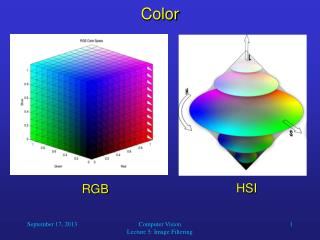DownloadDownload PresentationColor

# Color

Télécharger la présentation## Color

- - - - - - - - - - - - - - - - - - - - - - - - - - - E N D - - - - - - - - - - - - - - - - - - - - - - - - - - -
##### Presentation Transcript

1. RGB HSI Color Computer Vision Lecture 5: Image Filtering

2. Conversion from RGB to HSI • It is not too difficult to convert RGB values into HSI values to facilitate color processing in computer vision applications. • First of all, we normalize the range of the R, G, and B components to the interval from 0 to 1. • For example, for 24-bit color information, this can be done by dividing each value by 255. • Then we compute the intensity I as • I = 1/3*(R + G + B). • Obviously, intensity also ranges from 0 to 1. Computer Vision Lecture 5: Image Filtering

3. Conversion from RGB to HSI • Then we compute the values r, g, b that are independent of intensity: • r = R/(R + G + B) • g = G/(R + G + B) • b = B/(R + G + B) • When we consider the RGB cube, then all possible triples (r, g, b) lie on a triangle with corners (1, 0, 0), (0, 1, 0), and (0, 0, 1). • We could call this the rgb-subspace of our RGB cube. Computer Vision Lecture 5: Image Filtering

4. green p - w p = (r, g, b) pr - w H red (pr) w = (1/3, 1/3, 1/3) (white) blue Conversion from RGB to HSI • The hue is the angle H from vector pr – w to vector p – w. • The saturation is the distance from w to p relative to the distance from w to the fully saturated color of the same hue as p (on the edge of the triangle). Computer Vision Lecture 5: Image Filtering

5. Conversion from RGB to HSI • Then we have: Since w = (1/3, 1/3, 1/3): And since pr = (1, 0, 0): Computer Vision Lecture 5: Image Filtering

6. Conversion from RGB to HSI • We can also compute: With the above formulas, including those for deriving r, g, and b from R, G, and B, we can determine an equation for computing H directly from R, G, and B: Computer Vision Lecture 5: Image Filtering

7. Conversion from RGB to HSI Note that when we use the arccos function to compute H, arccos always gives you a value between 0 and 180 degrees. However, H can assume values between 0 and 360 degrees. If B > G, then H must be greater than 180 degrees. Therefore, if B > G, just compute H as before and then take (360 degrees – H) as the actual hue value. Computer Vision Lecture 5: Image Filtering

8. Conversion from RGB to HSI The saturation is the distance on the triangle in the rgb-subspace from white relative to the distance from white to the fully saturated color with the same hue. Fully saturated colors are on the edges of the triangle. The derivation of the formula for saturation S is very lengthy, so we will just take a look at the result: Computer Vision Lecture 5: Image Filtering

9. Limitations of RGB and HSI Using three individual wavelengths to represent color can never cover the entire visible range of colors: Computer Vision Lecture 5: Image Filtering

10. Limitations of any Color Representation It is important to note (again) that our perception of an object’s color does not only depend on the frequency spectrum emitted from the object’s location. It also depends on the spectra of other objects or regions in the visual field. This mechanism called color constancy allows us to assign a color to a given object that is invariant to shading or illumination of the scene by varying light sources. Computer Vision Lecture 5: Image Filtering

11. Limitations of any Color Representation Computer Vision Lecture 5: Image Filtering

12. Limitations of any Color Representation Computer Vision Lecture 5: Image Filtering

13. Let’s move on to… • Image Filtering Computer Vision Lecture 5: Image Filtering

14. Histogram Modification • A common and important filter operation is histogram modification. • Between any two stages of image processing, it often happens that the range of intensity values in our image is only a small proportion of the possible range. • This means that the contrast in the image is weaker than it would have to be. • It is then useful to modify the intensity histogram of the image. Computer Vision Lecture 5: Image Filtering

15. Histogram Modification • One possible method for this is image scaling: We simply expand the range [a, b] containing most of the intensities in the image to fill the entire range [z1, zk]. • This means that the value z of each pixel in the original image is mapped onto the value z’ in the scaled image in the following way: Notice that this method may leave gaps between bins in the resulting histogram. Computer Vision Lecture 5: Image Filtering